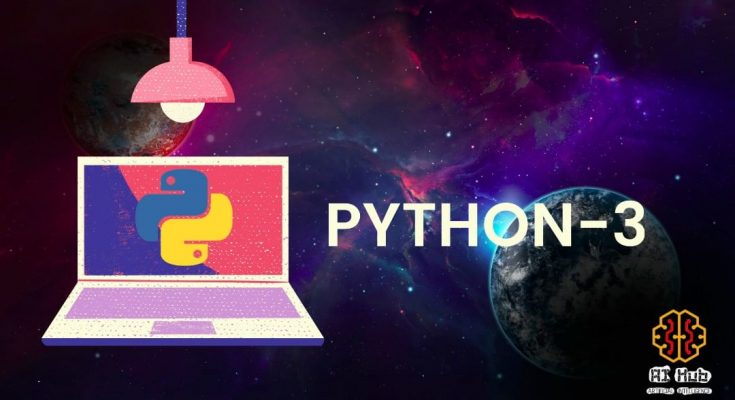# OPERATORS IN PYTHONIn the previous section, we learnt about various data types in python and respective methods. In this lesson onward we’ll dig into the semantics of the various operators in python. By the end of this section, you will have the detail knowledge about operators in python and their respective functions.

##### Python Operators

Operators are symbols which are used to perform operations on values and variables. We also define operators in python as the constructs which can manipulate the value of operands. The data items are referred as operands or arguments. We represent operators by keywords or special characters.

• Arithmetic operators
• Comparison operators
• Assignment Operators
• Logical Operators
• Bit wise Operators
• Membership Operators
• Identity Operators
##### Arithmetic operators

These operators in python are used to perform arithmetic operations like addition, subtraction, multiplication, division etc. Python implements seven basic binary arithmetic operators, two of which can double as unary operators. [ unary operators are those operators which operates on only one operand example: unary plus(+a) ]

##### EXAMPLES
``````>>> a = 6
>>> b = 4
>>> print('addition of a & b is ', a+b)
>>> print('subtraction of a & b is ', a-b)
>>> print('multiplication of a & b is ', a*b)
>>> print('division of a & b is ', a/b)
>>> print('Modulus of a & b is ', a%b)
>>> print('Floor division of a & b is ', a//b)
>>> print('b exponent of a  ', a**b)
>>> print('negation of a  is ', -a)
>>> print('unary plus of  is ', +a)``````
##### OUTPUT
``````addition of a & b is  10
subtraction of a & b is  2
multiplication of a & b is  24
division of a & b is  1.5
Modulus of a & b is  2
Floor division of a & b is  1
b exponent of a   1296
negation of a  is  -6
unary plus of  is  6``````

The floor division operator was added in Python 3; you should be aware if working in Python 2 that the standard division operator (/)acts like floor division for integers and like true division for floating-point numbers.

##### Projects with free Codes

This page is contributed by Diwas & Sunil . If you like AIHUB and would like to contribute, you can also write an article & mail your article to  itsaihub@gmail.com . See your articles appearing on AI HUB platform and help other AI Enthusiast.Highly motivated, strong drive with excellent interpersonal, communication, and team-building skills. Motivated to learn, grow and excel in Data Science, Artificial Intelligence, SEO & Digital Marketing

View all posts by Diwas Pandey →

### 2 Comments on “OPERATORS IN PYTHON”

1. Greetings! Very helpful advice within this post! It is the little changes
that produce the most significant changes. Thanks a lot
for sharing! adreamoftrains web hosting companies

2.web hosting reviews says:

Hello There. I found your blog using msn. This is an extremely well written article.